Next: Model Simulation and Image Up: Hidden Markov Random Field Previous: Markov Random Field Theory

## Hidden Markov Random Field Model

The concept of a hidden Markov random field model is derived from hidden Markov models (HMM), which are defined as stochastic processes generated by a Markov chain whose state sequence cannot be observed directly, only through a sequence of observations. Each observation is assumed to be a stochastic function of the state sequence. The underlying Markov chain changes its state according to a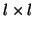transition probability matrix, where l is the number of states. HMMs have been applied successfully to speech recognition [26,30] and handwritten script recognition . Since original HMMs were designed as 1D Markov chains with first order neighbourhood systems, it can not directly be used in 2D/3D problems such as image segmentation. Here, we consider a special case of a HMM, in which the underlying stochastic process is a Markov random field (MRF), instead of a Markov chain, therefore not restricted to 1D. We refer to this special case as a hidden Markov random field (HMRF) model. Mathematically, an HMRF model is characterized by the following:
• Hidden Random Field (MRF) The Random field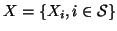is an underlying MRF assuming values in a finite state space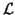with probability distribution (5). The state of X is unobservable.
• Observable Random Field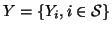is a random field with a finite state space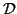. Given any particular configuration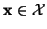, every Yi follows known conditional probability distribution p(yi|xi) of the same functional form, where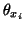is the involved parameters. This distribution is called the emission probability function and Y is also referred to as the emitted random field.
• Conditional Independence For any, the random variables Yi are conditional independent,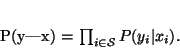(8)

Based on the above, we can write the joint probability of (X,Y) as

 P(y, x) = P(y|x)P(x) =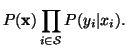According to the local characteristics of MRFs, the joint probability of any pair of (Xi, Yi), given Xi's neighbourhood configuration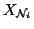, is: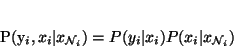(9)

Thus, we can compute the marginal probability distribution of Yi dependent on the parameter set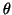(in this case, we treatas a random variable) and,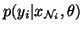=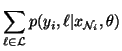=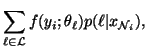(10)

where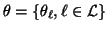. We call this the hidden Markov random field (HMRF) model. Note, the concept of an HMRF is different from that of an MRF in the sense that the former is defined with respect to a pair of random variable families (X,Y) while the latter is only defined with respect to X. More precisely, an HMRF model can be described by the following:
•- hidden MRF, with prior distribution p(x);
•- observable random field, with emission probability distribution p(yi|xi) for each yi;
•- the set of parameters involved in the above distributions.
If we assume the random variables Xi are independent of each other, which means that for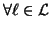and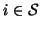, we have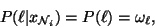then equation(10) reduces to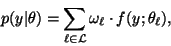which is the definition of the finite mixture model. Therefore a FM model is a degenerate special case of an HMRF model. It is obvious from the above that the fundamental difference between the FM model and the HMRF model lies in their different spatial properties. The FM model is spatially independent whereas the HMRF model may be spatially dependent. Therefore, the HMRF model is more flexible for image modelling in the sense that it has the ability to encode both the statistical and spatial properties of an image. With a Gaussian emission distribution, the FM model is usually known as the finite Gaussian Mixture (FGM) or finite normal mixture (FNM) model. More specifically, the observable random variables have the following density function: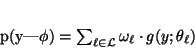(11)

where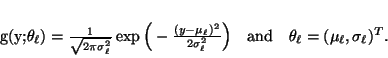(12)

Similarly, an HMRF model with a Gaussian emission distribution can be specified as: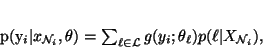(13)

where g and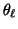are defined as in (12). We refer to this type of HMRF as the Gaussian hidden Markov random field (GHMRF) model.Next: Model Simulation and Image Up: Hidden Markov Random Field Previous: Markov Random Field Theory
Yongyue Zhang
2000-05-11